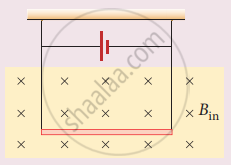Tamil Nadu Board of Secondary EducationHSC Science Class 12th

# A conductor of linear mass density 0.2 g m1 suspended by two flexible wire as shown in figure. Suppose the tension in the supporting wires is zero when it is kept inside the magnetic field of - Physics

Numerical

A conductor of linear mass density 0.2 g m1 suspended by two flexible wire as shown in figure. Suppose the tension in the supporting wires is zero when it is kept inside the magnetic field of I T whose direction is into the page. Compute the current inside the conductor and also the direction for the current. Assume g = 10 m s-2.#### Solution

Downward force, F = mg

Linear mass density, m/l = 0.2 gm-1

m/l = 0.2 x 10-3 kg m-1

m = (0.2 x 10-3 x l) kg m-1

F = (0.2 x 10-3 x l x 10) N

Upward magnetic force acting on the wire

F = BIl

0.2 x 10-3 x l x 10 = 1 x I x l

I = 2 x 10-3

I = 2 m A

Concept: Magnetic Effects of Current
Is there an error in this question or solution?

#### APPEARS IN

Tamil Nadu Board Samacheer Kalvi Class 12th Physics Volume 1 and 2 Answers Guide
Chapter 3 Magnetism and magnetic effects of electric current
Evaluation | Q IV. 2. | Page 192
Share